Instructions

For the following questions answer them individually

Question 1

# A tennis ball is dropped on a horizontal smooth surface. It bounces back to its original position after hitting the surface. The force on the ball during the collision is proportional to the length of compression of the ball. Which one of the following sketches describes the variation of its kinetic energy K with time t most appropriately? The figures are only illustrative and not to the scale.Question 2

# A wire, which passes through the hole in a small bead, is bent in the form of quarter of a circle. The wire is fixed vertically on ground as shown in the figure. The bead is released from near the top of the wire and it slides along the wire without friction. As the bead moves from A to B, the force it applies on the wire is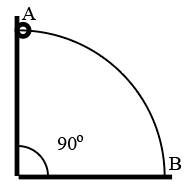Question 3

# During an experiment with ametre bridge, the galvanometer shows a null point when the jockey is pressed at 40.0 cm using a standard resistance of $$90 Ω$$,as shown in the figure. The least count of the scale used in the metre bridge is 1 mm. The unknown resistance is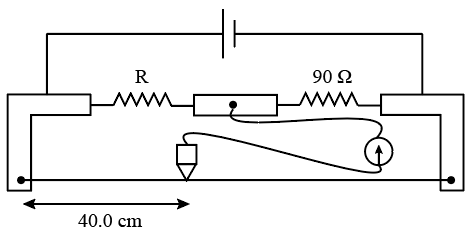Question 4

# Charges Q, 2Q and 4Q are uniformly distributed in three dielectric solid spheres 1, 2 and 3 of radii R/2, R and 2R, respectively, as shown in figure. If magnitudes of the electric fields at point P at a distance R from the center of spheres 1, 2 and 3 are $$E_1 , E_2$$ and $$E_3$$ respectively, then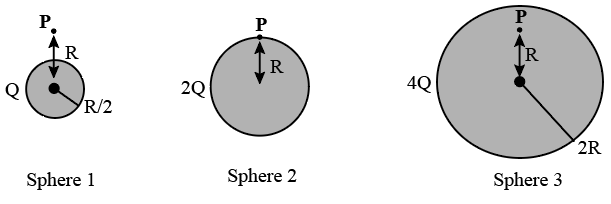Question 5

# A point source S is placed at the bottom of a transparent block of height 10 mm and refractive index 2.72. It is immersed in a lower refractive index liquid as shown in the figure. It is found that the light emerging from the block to the liquid forms a circular bright spot of diameter 11.54 mm on the top of the block. The refractive index of the liquid is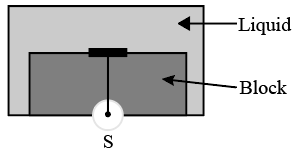Question 6

# Parallel rays of light of intensity $$I = 912 Wm^{-2}$$ are incident on a spherical black body kept in surroundings of temperature 300 K. Take Stefan-Boltzmann constant $$\sigma = 5.7 \times 10^{-8} Wm^{-2} K^{-4}$$ and assume that the energy exchange with the surroundingsis only through radiation. The final steady state temperature of the black body is close toQuestion 7

# A metal surface is illuminated by light of two different wavelengths 248 nm and 310 nm. The maximum speeds of the photoelectrons corresponding to these wavelengths are $$u_1$$ and $$u_2$$,respectively. If the ratio $$u_1 : u_2 = 2 : 1$$ and hc = 1240 eV nm, the work function of the metal is nearlyQuestion 8

# If $$\lambda_{Cu}$$ is the wavelength of $$K_{\alpha}$$ X-ray line of copper (atomic number 29) and $$\lambda_{Mo}$$ is the wavelength of the $$K_{\alpha}$$ X-ray line of molybdenum (atomic number 42), then the ratio $$\frac{\lambda_{Cu}}{\lambda_{Mo}}$$is close toQuestion 9

# A planet of radius $$R = \frac{1}{10} \times$$ (radius of Earth) has the same mass density as Earth. Scientists dig a well of depth $$\frac{R}{5}$$ on it and lower a wire of the same length and of linear mass density $$10^{-3} kgm{-1}$$ into it. If the wire is not touching anywhere, the force applied at the top of the wire by a person holding it in place is (take the radius of Earth $$= 6 \times 10^6 m$$ and the acceleration due to gravity on Earth is $$10 ms^{-2}$$)Question 10

# A glass capillary tube is of the shape of a truncated cone with an apex angle $$\alpha$$ so that its two ends have cross sections of different radii. When dipped in water vertically, water rises in it to a height h, where the radius of its cross section is b. If the surface tension of water is S, its density is $$\rho$$, and its contact angle with glass is $$\theta$$, the value of h will be (g is the acceleration due to gravity)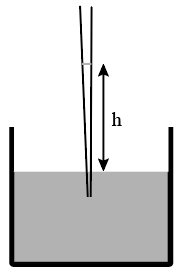OR